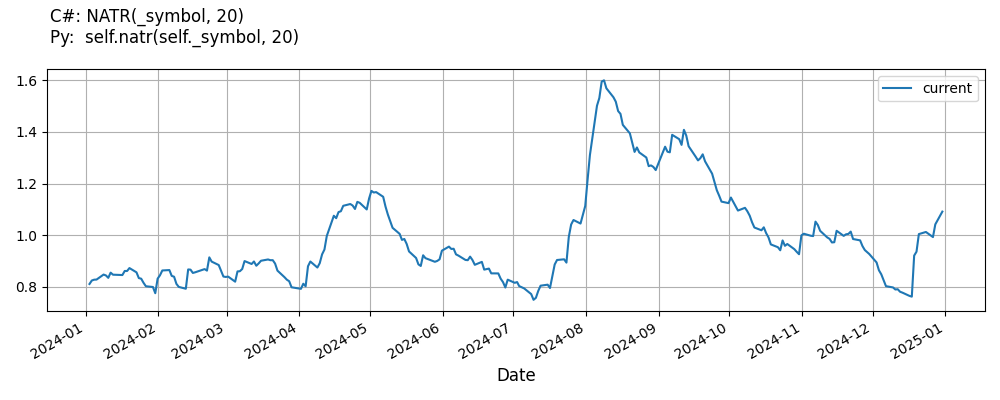# Supported Indicators

## Normalized Average True Range

### Introduction

This indicator computes the Normalized Average True Range (NATR). The Normalized Average True Range is calculated with the following formula: NATR = (ATR(period) / Close) * 100

To view the implementation of this indicator, see the LEAN GitHub repository.

### Using NATR Indicator

To create an automatic indicators for NormalizedAverageTrueRange, call the NATR helper method from the QCAlgorithm class. The NATR method creates a NormalizedAverageTrueRange object, hooks it up for automatic updates, and returns it so you can used it in your algorithm. In most cases, you should call the helper method in the Initialize method.

public class NormalizedAverageTrueRangeAlgorithm : QCAlgorithm
{
private Symbol _symbol;
private NormalizedAverageTrueRange _natr;

public override void Initialize()
{
_natr = NATR(_symbol, 20);
}

public override void OnData(Slice data)
{
{
// The current value of _natr is represented by itself (_natr)
// or _natr.Current.Value
Plot("NormalizedAverageTrueRange", "natr", _natr);

}
}
}
class NormalizedAverageTrueRangeAlgorithm(QCAlgorithm):
def Initialize(self) -> None:
self.natr = self.NATR(self.symbol, 20)

def OnData(self, slice: Slice) -> None:
# The current value of self.natr is represented by self.natr.Current.Value
self.Plot("NormalizedAverageTrueRange", "natr", self.natr.Current.Value)



The following reference table describes the NATR method:

### NATR()1/1

            NormalizedAverageTrueRange QuantConnect.Algorithm.QCAlgorithm.NATR (
Symbol                                symbol,
Int32                                 period,
*Nullable<Resolution>           resolution,
*Func<IBaseData, IBaseDataBar>  selector
)


Creates a new NormalizedAverageTrueRange indicator.

If you don't provide a resolution, it defaults to the security resolution. If you provide a resolution, it must be greater than or equal to the resolution of the security. For instance, if you subscribe to hourly data for a security, you should update its indicator with data that spans 1 hour or longer.

You can manually create a NormalizedAverageTrueRange indicator, so it doesn't automatically update. Manual indicators let you update their values with any data you choose.

Updating your indicator manually enables you to control when the indicator is updated and what data you use to update it. To manually update the indicator, call the Update method with a TradeBar, or QuoteBar. The indicator will only be ready after you prime it with enough data.

public class NormalizedAverageTrueRangeAlgorithm : QCAlgorithm
{
private Symbol _symbol;
private NormalizedAverageTrueRange _natr;

public override void Initialize()
{
_natr = new NormalizedAverageTrueRange(20);
}

public override void OnData(Slice data)
{
if (data.Bars.TryGeValue(_symbol, out var bar))
{
_natr.Update(bar);
}

{
// The current value of _natr is represented by itself (_natr)
// or _natr.Current.Value
Plot("NormalizedAverageTrueRange", "natr", _natr);

}
}
}
class NormalizedAverageTrueRangeAlgorithm(QCAlgorithm):
def Initialize(self) -> None:
self.natr = NormalizedAverageTrueRange(20)

def OnData(self, slice: Slice) -> None:
bar = slice.Bars.get(self.symbol)
if bar:
self.natr.Update(bar)

# The current value of self.natr is represented by self.natr.Current.Value
self.Plot("NormalizedAverageTrueRange", "natr", self.natr.Current.Value)



To register a manual indicator for automatic updates with the security data, call the RegisterIndicator method.

public class NormalizedAverageTrueRangeAlgorithm : QCAlgorithm
{
private Symbol _symbol;
private NormalizedAverageTrueRange _natr;

public override void Initialize()
{
_natr = new NormalizedAverageTrueRange(20);
RegisterIndicator(_symbol, _natr, Resolution.Daily);
}

public override void OnData(Slice data)
{
{
// The current value of _natr is represented by itself (_natr)
// or _natr.Current.Value
Plot("NormalizedAverageTrueRange", "natr", _natr);

}
}
}
class NormalizedAverageTrueRangeAlgorithm(QCAlgorithm):
def Initialize(self) -> None:
self.natr = NormalizedAverageTrueRange(20)
self.RegisterIndicator(self.symbol, self.natr, Resolution.Daily)

def OnData(self, slice: Slice) -> None:
# The current value of self.natr is represented by self.natr.Current.Value
self.Plot("NormalizedAverageTrueRange", "natr", self.natr.Current.Value)



The following reference table describes the NormalizedAverageTrueRange constructor:

### NormalizedAverageTrueRange()1/2

            NormalizedAverageTrueRange QuantConnect.Indicators.NormalizedAverageTrueRange (
string  name,
int     period
)


Initializes a new instance of the NormalizedAverageTrueRange class using the specified name and period.

### NormalizedAverageTrueRange()2/2

            NormalizedAverageTrueRange QuantConnect.Indicators.NormalizedAverageTrueRange (
int  period
)


Initializes a new instance of the NormalizedAverageTrueRange class using the specified period.

### Visualization

The following image shows plot values of selected properties of NormalizedAverageTrueRange using the plotly library.You can also see our Videos. You can also get in touch with us via Discord.